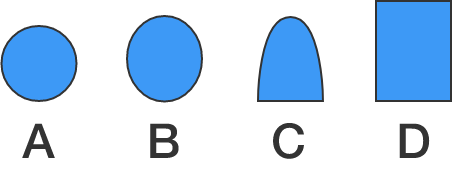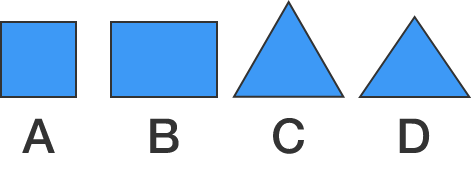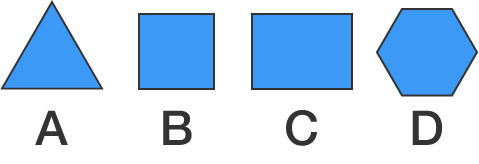### 3D Geometry

A cross-section of a 3D figure is the intersection of the figure with a plane. You can adjust the plane below by moving the sliders.

Which of the following shapes could not be a cross-section of a cylinder?# Cuts Through Shapes

Which of the following shapes could not be a cross section of a cube?# Cuts Through Shapes

We'll be working with 3D figures in this course, and one way we can better understand them is by relating them with familiar 2D figures. It can be useful to think of cross sections of a 3D solid as images from an MRI machine.

MRIs take images of thin slices as they scan through a solid. Hitting the "play" button or moving the slider under the figure above lets you see the scanning plane pass through the cube, while the play button and slider below show how the cross sections change as the plane moves.

# Cuts Through Shapes

Suppose we have a plane oriented so that its intersection with a cube makes an equilateral triangle. As you move the slider in the diagram below, the plane keeps the same orientation.

If the plane kept moving in the same direction until it divided the cube precisely in half, what shape would the cross-section be?

# Cuts Through Shapes

Here, we see how the cross-sections from the previous question look as the plane moves through the cube from one corner to another.

# Cuts Through Shapes

Which solid might have this sequence of cross sections as a plane passes through it?

# Cuts Through Shapes

A hexagonal prism, a cylinder, and a cube are standing next to each other (in some order). The visualization below shows how the cross-sections change as a plane scans through all three simultaneously.

What is the order of the solids, from left to right?

# Cuts Through Shapes

If you've made a slice through a cube that divides the cube's volume exactly in half, what could the slice's cross-section not look like?×